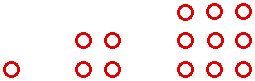# Square Numbers

Consider the pattern formed by these dots.The number used to describe each “square” is called a square number. Given a number, can you arrange that many dots into a square? If you can, you have identified a square number. The figure above shows the first three square numbers, 1, 4, and 9. How can you make the next square number from parts of the third square number? Generalize your work. What is the sum of the first n square numbers?